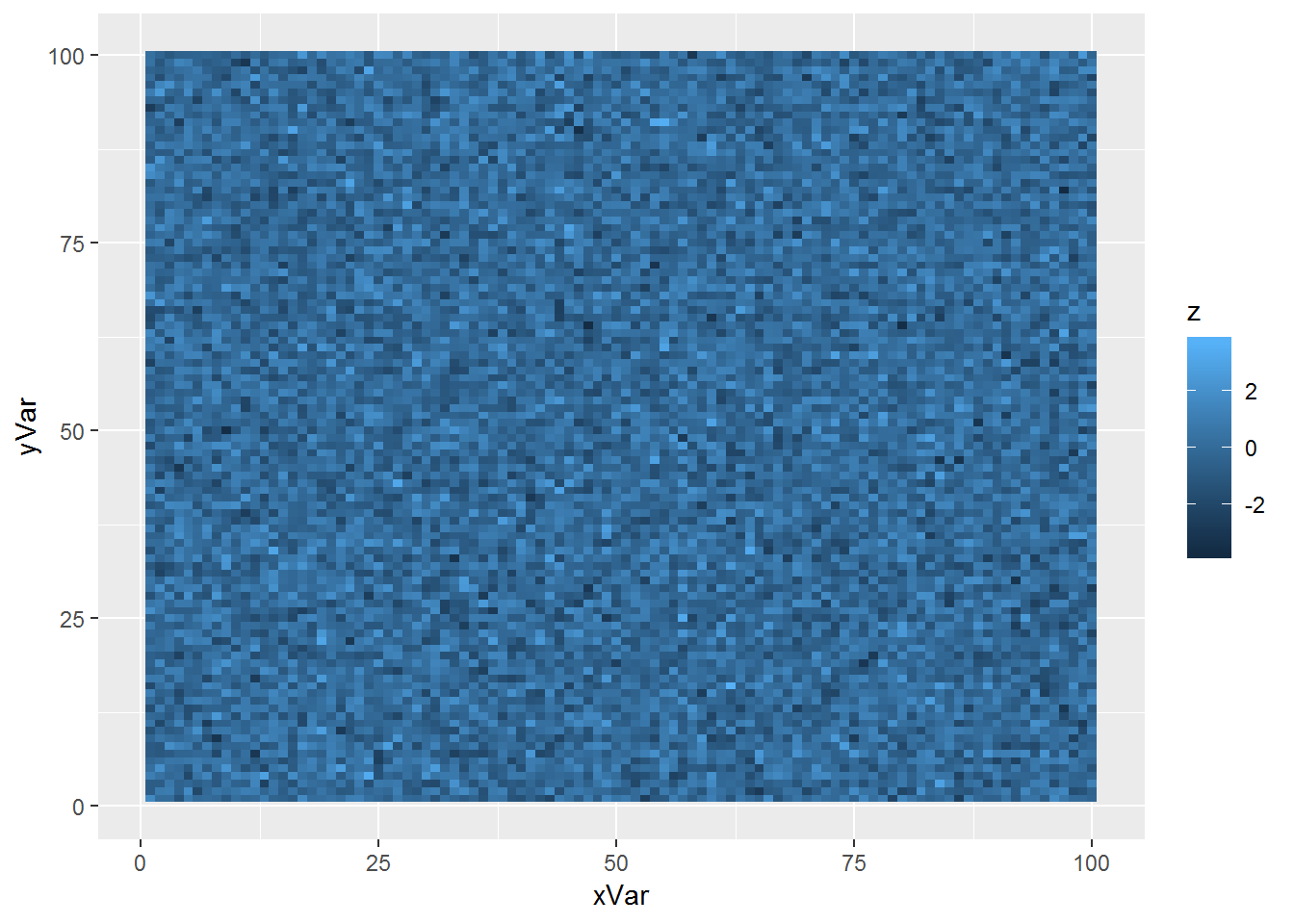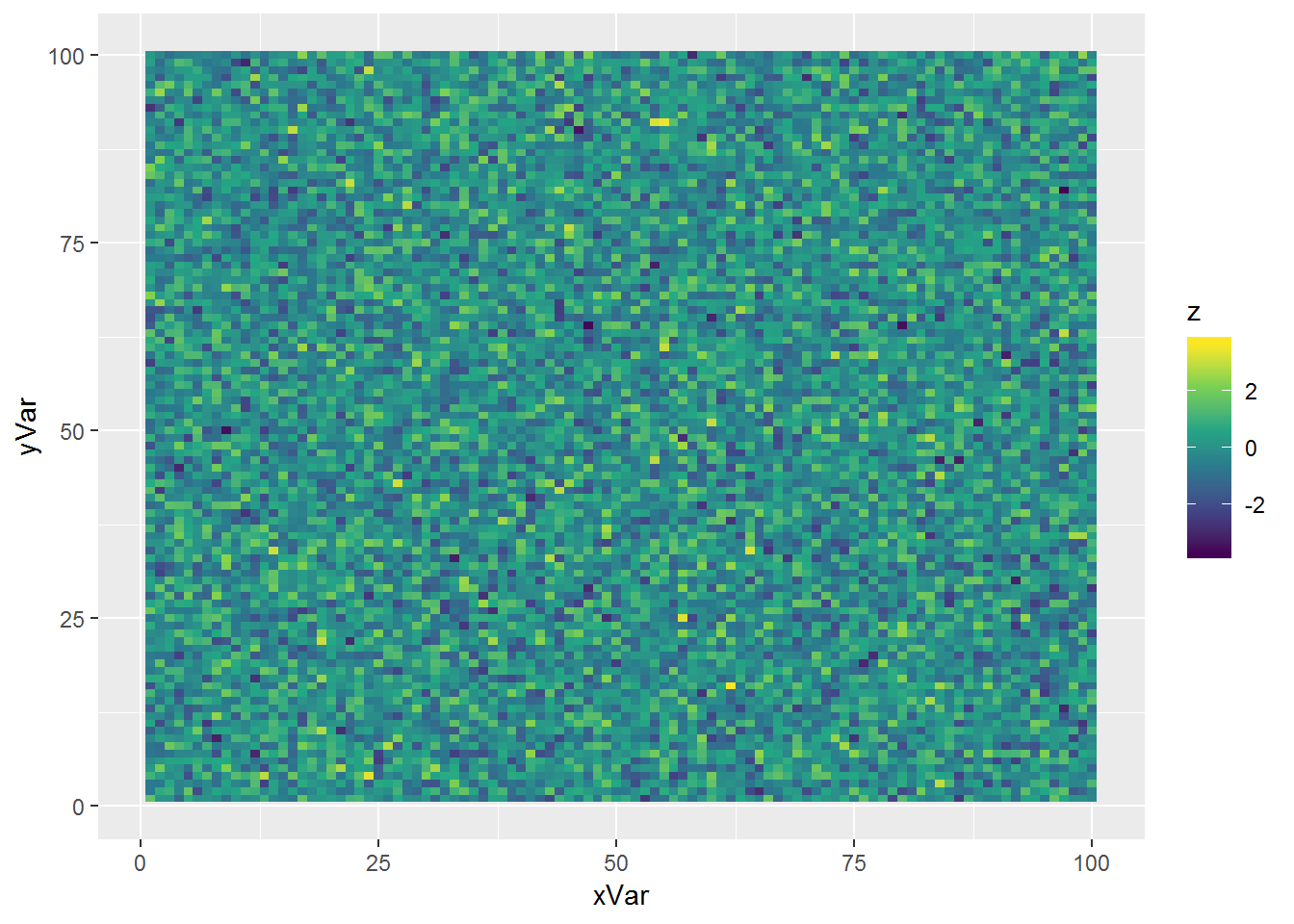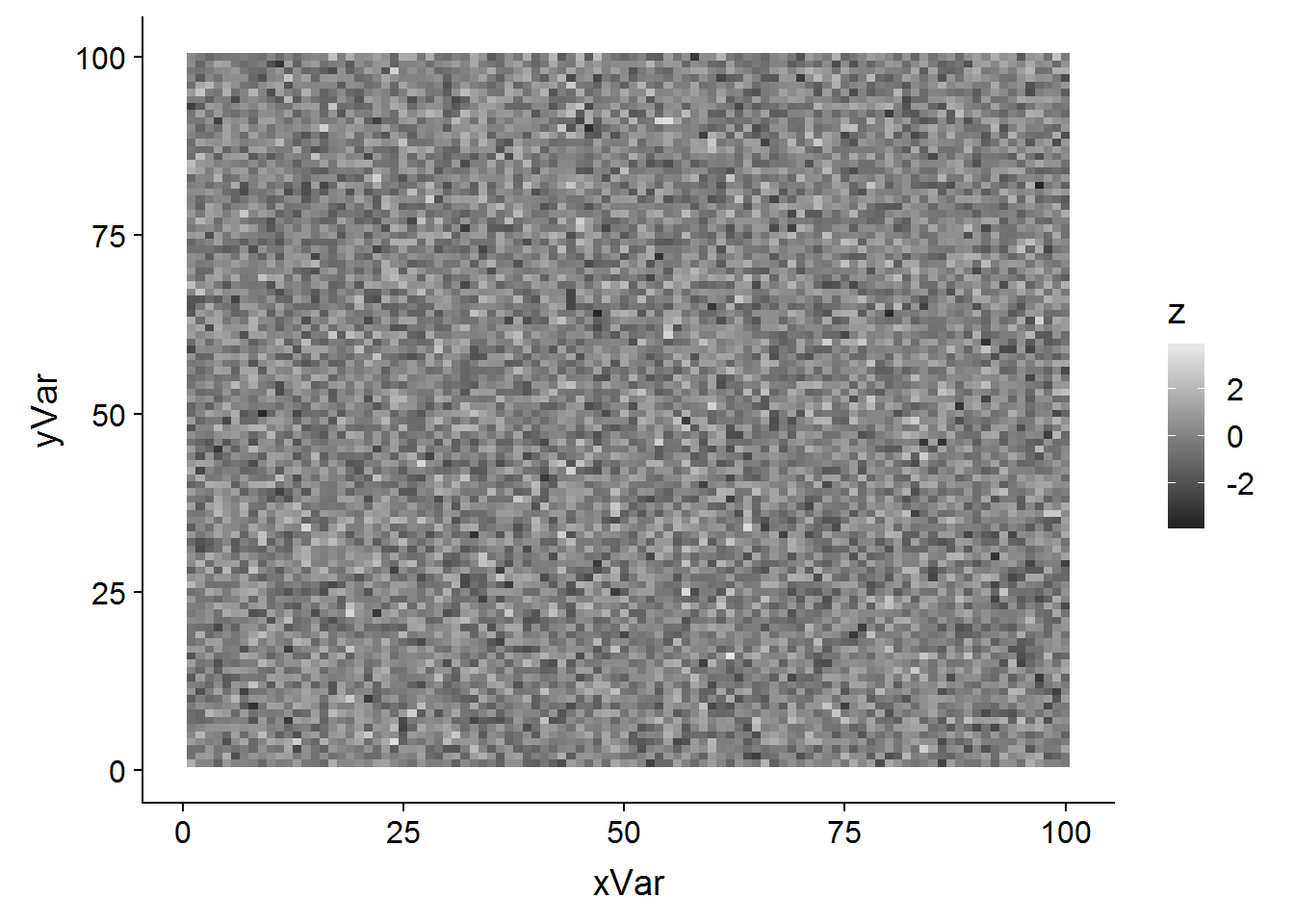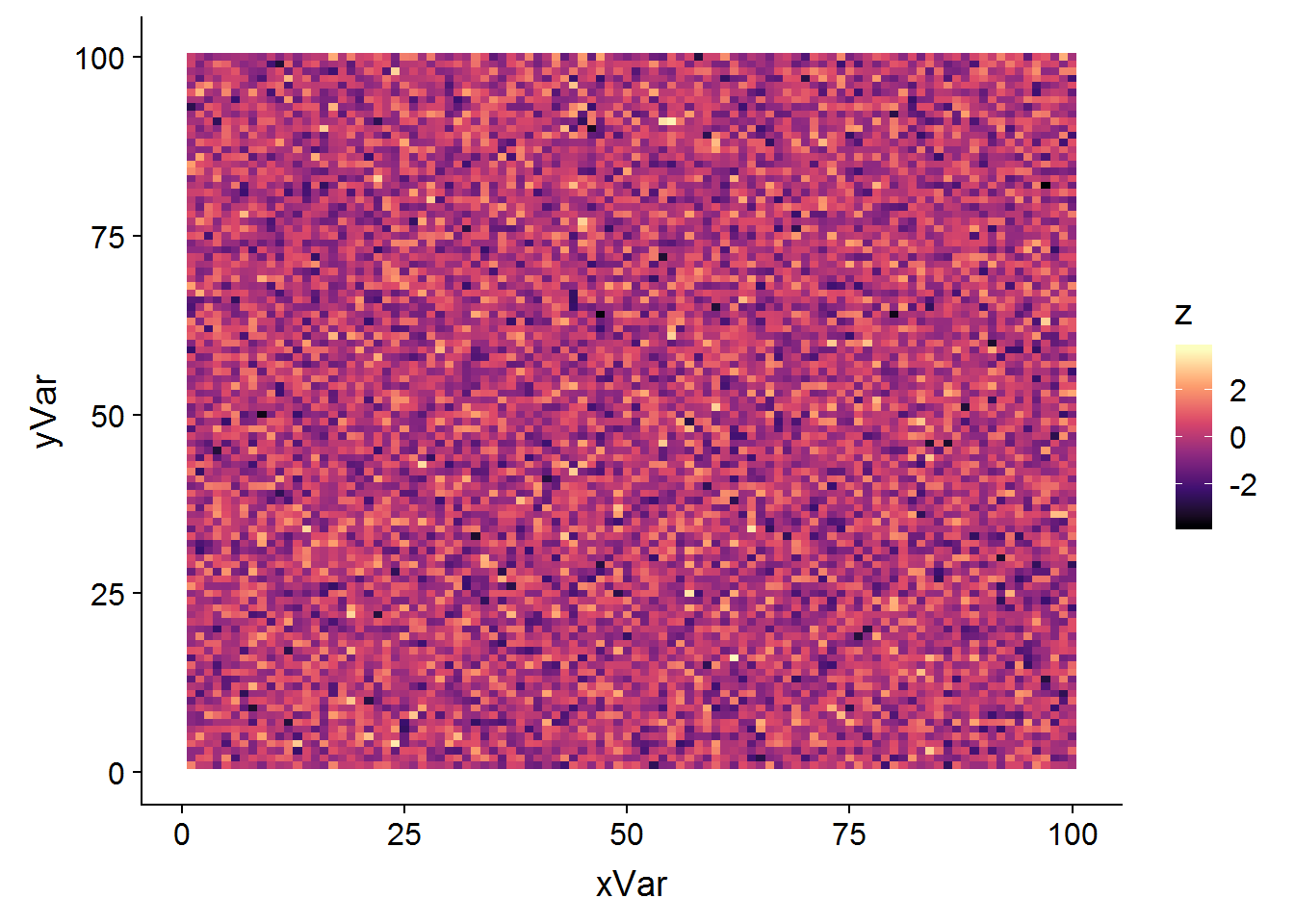R has just incorporated the `viridis` palette for heat maps. The advantages are: - a continuous blue to yellow scale that renders detail much more clearly than other palettes - the viridis palette is visible to those with color blindness - the viridis palette translates readily to a well-differentiated grey-scale.

https://www.r-bloggers.com/ggplot2-welcome-viridis/

I will illustrate its power for a heat map applied to a random normal variate. On average, the colors should not change across this heat map, but a good color scale will differentiate fine-scale peaks and valleys in the color landscape.

``````library(ggplot2)
library(TeachingDemos)
char2seed("Ozark")

# first create the data frame
dFrame <- expand.grid(xVar=1:100,yVar=1:100)
dFrame\$z <- rnorm(10000)``````

We start by using the default color palette for visualizing the heat map:

``````p1 <- ggplot(data=dFrame,aes(x=xVar,y=yVar)) +
geom_tile(aes(fill=z))
print(p1)``````Looks OK, but a bit monochrome. Now with the built-in `viridis` color scale:

``````p2 <- ggplot(data=dFrame,aes(x=xVar,y=yVar)) +
geom_tile(aes(fill=z)) +
scale_fill_viridis_c()
print(p2)``````This makes it much easier to pinpoint the outliers at both extremes.

Here is some excellent code from Lauren Ash for converting this to a grayscale plot:

``````library(colorblindr) # devtools::install_github("clauswilke/colorblindr")
library(colorspace) # install.packages("colorspace", repos = "http://R-Forge.R-project.org") --- colorblindr requires the development version
# this also installs cowplot
library(cowplot)
p3 <- ggplot(data=dFrame,aes(x=xVar,y=yVar)) +
geom_tile(aes(fill=z)) +
scale_fill_viridis_c()
p3des<-edit_colors(p3, desaturate)
ggdraw(p3des)``````Finally, there are some options for other scales that will give a more traditional heat map scale, but with the same features as the viridis scale:

``````p4 <- ggplot(data=dFrame,aes(x=xVar,y=yVar)) +
geom_tile(aes(fill=z))
p4 + scale_fill_viridis_c(option="magma") # "A" option````````p4 + scale_fill_viridis_c(option="inferno") # "B" option``